## Fourier transforms front page

### Quick description

The Fourier transform is a fundamental tool in many parts of mathematics. This is even more so when one looks at various natural generalizations of it. This article contains brief descriptions of the Fourier transform in various contexts and links to articles about its use.

### Prerequisites

Basic analysis, complex numbers.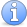Incomplete This article is incomplete. This article is incomplete in obvious ways.

### Different kinds of Fourier transform

Periodic functions and functions defined on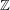. Let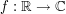be a function such that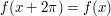for every. Then theth Fourier coefficient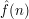is given by the formula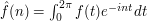. The function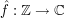is called the Fourier transform of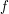. Periodic functions are naturally thought of as functions defined on the circle. If we write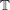for the unit circle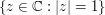and have a function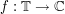, then the formula forbecomes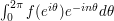.

In the other direction, let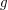be a function fromto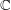. We can create a periodic function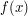by defining it to equal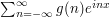. Under some circumstances, and with suitable notions of convergence, one can show that this inverts the previous operation: that is, the sum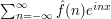converges to the function. If we expressas a function defined on, then this says that we can writeas a doubly infinite power series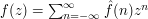, defined when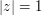.

Functions defined on the group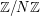of integers mod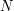. Letbe a function fromto. Write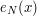for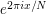. Then the discrete Fourier transform ofis the function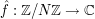given by the formula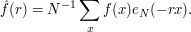(There are various alternative conventions for the precise definition here, but they all have the same important properties.) The discrete Fourier transform can be inverted as follows: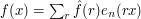.

Functions defined from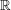to. In this case, the Fourier transform takes a functiondefined onto another function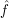defined onby the formula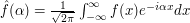. (Once again, there are several other conventions for the precise definition, which differ in inessential ways from the one we have chosen here.) For suitable functionsthis can be inverted as follows: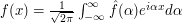.

Functions defined on finite Abelian groups. The Fourier transform for functions defined onis a special case of an important abstract definition of the Fourier function for functions defined on a finite Abelian group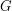. A character onis defined to be a homomorphism fromto the multiplicative group of non-zero complex numbers (which has to take values of modulus 1, so it can also be defined as a homomorphism to the unit circle in). It can be shown that the characters onform an orthonormal basis for the space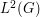of functions fromtowith the inner product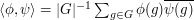. The Fourier expansion of a function is just its expansion in this basis. More concretely, if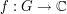and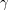is a character, then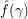is defined to be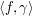, and we then have the inversion formula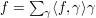, where the sum is over all characters. This gives us the definition we had earlier for functions defined on, except that we have to identify the character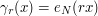with the element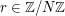.

Functions defined on locally compact Abelian groups. As the examples ofandshow, an Abelian groupdoes not have to be finite for it to be possible to define a Fourier transform for functions fromto. Indeed, we can use more or less the same definition and try to expand a function in terms of characters. However, whenis infinite, one does not normally take arbitrary characters: rather, the group usually has a topological structure and one asks for characters that are continuous. For instance, the continuous characters defined onare precisely the functions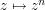with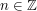, and that explains in an abstract way the definition of the Fourier transform for functions defined on.

There is some subtlety about what it means to decompose a function into characters, as the example of functions defined onshows. There, the characters are functions of the form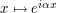with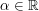. In a sense, we writeas a linear combination of characters, but the "linear combination" is an integral rather than a sum.

Fourier transforms of generalized functions. The Dirac delta function is an example of an object that is not in fact a function, but which has a Fourier transform: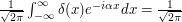for every, so its Fourier transform is a constant function. The inverse Fourier transform of the constant function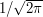is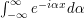, which can be interpreted aswhen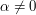andwhen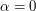, so we get something delta-function like. This vague idea can be made rigorous using the theory of distributions. In general, there are many objects, such as distributions and measures, for which one can usefully define Fourier transforms.

### Basic facts about the Fourier transform

To be included: Parseval/Plancherel identity, inversion formulae, convolution identities.

### Fourier transforms of measures

We need a -Fourier transforms of measures and a -Fourier transforms of distributions or you think it should be under the same title? I believe that Fourier transforms of measures deserve a special heading here. But I don't know where to start really. Trying to define Fourier transforms of measures in full generality might be confusing. I would start on the real line or the circle to make things more concrete and simple. Then I would go on defining Fourier transforms of measures in the Euclidean space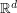. I guess some special section should be devoted to measures supported on sub-manifolds ofand there should be a chain that connects to curvature and oscillatory integrals. Also a 'Parent' of this article should be '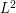estimates' but I don't know if there is such an article yet.

yannis